# Find the Maximum Sum of i*arr[i] Among all Possible Rotations of an Array

## Introduction

How often do you encounter problems that involve maximizing the value of a given function? It seems complicated to many people, but if one tends to analyze the functions properly, it won’t be too hard to figure out how to maximize it.The problem of finding the maximum sum of i*arr[i] among all possible rotations on a given array is a similar maximization problem.

Let’s look at the problem statement once. So we are given an array of integers. We need to find the maximum sum of i*arr[i], where i is the index, given that you can rotate the array any number of times.

Let’s look at an example where we will get more clarity of the problem.

Let’s say, we have an array of size N = 4,

Let’s say, we have an array of size N = 4,

and array is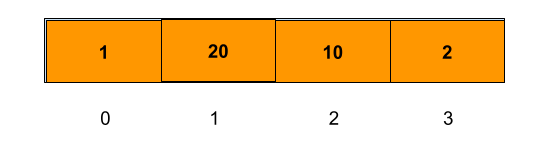Now let’s see all possible rotations and calculate the sum of i*arr[i] in each rotation.

How many rotations are possible?

We can make at most N rotations including the initial array to get a different array every time in general. Because if more than N rotations are considered, then we will be reconsidering already considered rotations.

1. Rotating for the first time, the array will become as :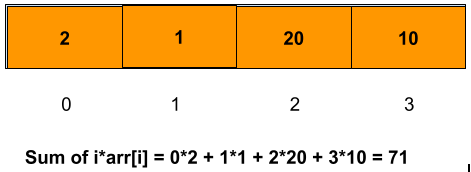2. Rotating for the second time, the array will become as :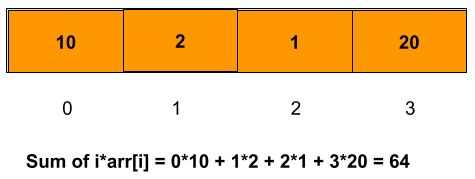3. Rotating for the third time, the array will become as :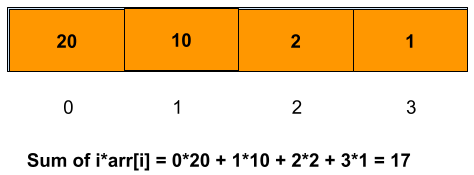4. Rotating for the fourth time, the array will become as :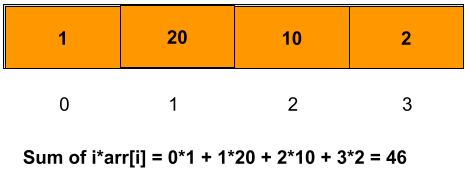From the four rotations, which rotation returns the maximum sum of i*arr[i]?

It is after the first rotation that the value is maximum, which is 71.

I hope you got the essence of the problem from the above example. If not, then no worries, now we will discuss the approach.

## Approach

Let’s look at the most trivial approach that comes to our mind first.

Calculating the sum of i*arr[i] in each rotation and maximize it.

So the approach becomes simple.

1. Calculate the value of the sum of i*arr[i].
2. Maximize the sum, and rotate the array.
3. Repeat the process until the number of rotations is not greater than N.

NOTE: if the number of rotations R is greater than N, then R should be taken as N only as the array after R rotations is the same as after R%N rotations.

Don’t worry about the time complexity here; we will have a look at it later on.

## PseudoCode

#Assume there is a function sumOfProducts(arr, n) that computes sum of i*arr[i].

Algorithm

___________________________________________________________________

procedure maxSumOfProducts(arr, n):

___________________________________________________________________

1. maxSum ←  -∞                # assume answer to be -inf

2. rotations ← 0          # to keep track of rotations

3. while rotations < n do     # run till n rotations

4. rotateArray(arr, n)   # rotate the array

5. rotatedArraySum ←  sumOfProducts(arr, n) #compute the sum i*arr[i]

6. if  rotatedArraySum > maxSum do   #update the maximum sum

7.         maxSum ←  rotatedArraySum

8.         end if

9.           rotations ← rotations + 1 # increment the count of rotations

10. end while

11.      return maxSum #return the maximum sum.

12.end procedure

___________________________________________________________________

## CODE IN C++

Output

### Time Complexity

O(n2) because we are traversing the array again in each rotation for computing the sum of i*arr[i], which takes O(n) time. For n rotations will take O(n2) time to compute the maximum sum of i*arr[i] using the above algorithm.

### Space complexity

O(n), as we are rotating the array.

Rotating the array repeatedly and computing the sum of products takes a lot of time as it does redundant work. So can we come up with some efficient approach that does the same job in less time?So, let's discuss how we can optimize the solution for the problem.

## Approach to a Time-efficient Solution

Now the very first question is, where are we consuming time?

(Note: It’s imperative to understand and find the root cause of the problem before directly jumping on to its solution.)

We are taking time to rotate the array repetitively and computing the sum of products again and again.

Now the question arises which repetition should we remove first? Should we remove any repetitive work, i.e., either computing the sum of products or avoiding rotating the array.

We will have to calculate the sum for every rotation, so if we can somehow sum up each rotation of the array efficiently, we can significantly reduce the repetitive work.

To compute the sum efficiently, we need to observe how the sum changes with each rotation.

Let’s say after r rotations, we have the array, which looks like:

Ar = {a0 , a1 …..,   a n-2 , a n-1 }

S = 0*a0 + 1*a1 +.. + (n-2)*a n-2 + (n-1)*a n-1

Now, after r+1 rotations, we have the array which looks like,

Ar+1 = {a n-1 , a0 …..   a n-3 , a n-2 }

Sr+1  = 0*a n-1 + 1*a0 +.. + (n-2)*a n-3 + (n-1)*a n-2

Can we observe some relation between the terms S r+1 and S ?

Let’s try to find some relation between the 2 terms. So if we are given a sum of rth rotation and want to compute the (r+1)th rotation, we will have to add or subtract some term to it.

Let’s say,

This is because it will not be correct if we simply write C = 1 initially. We can put the value of C later on according to our convenience, which simplifies our computation, and we can get to a good relationship between them.

Note that we also want to compute X, so the logical thing is to subtract

S r+1  and C*Sr.

S r+1 C*S= (0*a n-1 + 1*a0 +.. + (n-2)*a n-3 + (n-1)*an-2) - C*(0*a0 + 1*a1 +.. + (n-2)*a n-2 + (n-1)*a n-1

S r+1 C*S= ((1-0*C)*a0 + (2-1*C)a1+.. (n-1 -(n-2)*C)an-2 + (0 - (n-1)*C)an-1)

Now, we can put C = 1 because X is also dependent on C, so removing the term C from our equation will make it easier to understand.

S r+1 Sr = (a0 + (2-1)a..+.. (n-1 -n+2)an-2 + (-(n-1))an-1)

= a0 + a1 + …. + an-2 + (1-n)*an-1

a0 + a1 + …. + an-2 + a n-1 - n*a n-1

= sumOfArray - n*a n-1

Another thing to look at is that n-1 will keep changing with each rotation, so we must take care of that.

After rth rotation, n-1 = a n-r as the previous element shifts by one position with each rotation. Hence we derived the value of n-1.

Therefore, S r+1 Sr =  sumOfArray - n*a n-r

Now, this simplifies our result, i.e., we just need to compute the sum of the array once, and the rest is adding the subtraction result to Sr

Now for each rotation, we have the sum using the above result.

### Algorithm(space and time optimized)

1. Compute the sum of the array initially.
2. For each rotation, compute the sum using the above result
3. Maximize the result and repeat the process until all the rotations are covered.

I guess the algorithm says it all. There’s no need for giving another pseudoCode because all the techniques are pretty familiar to us. Hence we can jump onto the coding part now. (Don’t worry, the code part will be self-explanatory).

## CODE IN C++(Space and Time Optimized)

Output

### Time Complexity

O(n) because we are computing the sum of each rotation in O(1) time, and the only time taken is to calculate the sum of the array once and iterating over all rotations. Hence the time complexity is O(n).

### Space complexity

O(1), as no extra auxiliary space is used.

How to find the element at a given index after r rotations?

Answer) Let r be the number of rotations and n be the size of the array. Let i_old be the index before rotation and i_after be the index after rotation. Then we have

How do you find the maximum sum of an array?

Answer) The maximum sum of an array is a very common problem that is solved using Kadane’s Algorithm. To know more about Kadane’s Algorithm, refer here.

How do you find the minimum sum of an array?

Answer) The minimum sum of an array is a common application of Kadane’s algorithm where we minimize the result at each iteration.

## Key Takeaways

This article taught us how to maximize the sum of i*arr[i] by approaching the problem using a brute force approach to the most optimal approach finally. We discussed their implementation using an iterative method using illustrations, through pseudocode, and then proper code. We hope you were able to take away critical techniques like analyzing problems by writing some mathematical formulations and manipulating them, which simplifies the results to a greater extent.

Now, we recommend you practice problem sets based on maximization problems to master your fundamentals. You can get a wide range of questions similar to the problem maximum sum of i*arr[i] on CodeStudio